# Universal Gate: NAND and NOR Gate as Universal Gates

Contents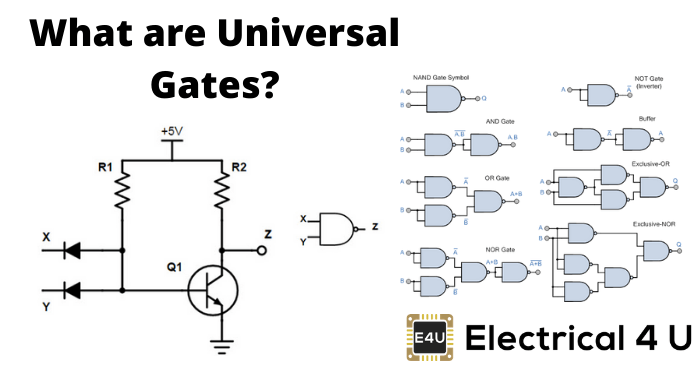## What are Universal Gates?

A universal gate is a logic gate which can implement any Boolean function without the need to use any other type of logic gate. The NOR gate and NAND gate are universal gates. This means that you can create any logical Boolean expression using only NOR gates or only NAND gates.

In practice, this is advantageous since NOR and NAND gates are economical and easier to fabricate than other logic gates. So much so that an AND gate is typically implemented as a NAND gate followed by an inverter (not the other way around)! Similarly, an OR gate is typically realised as a NOR gate followed by an inverter.

Other logical gates – such as AND gates, NOT gates and OR gates – do not have this property of universality. Note that combined these three logic gates can implement all of the possible Boolean switching functions, but not individually. Hence unlike the NOR and NAND logic gates, they are not classified as universal gates.

If you which to play around with these universal gates as part of an electronics project, many of the best Arduino starter kits contain these universal NOR and NAND gates.

Now we will look at the operation of NOR gates and NAND gates as universal gates.

## NAND Gate As A Universal Gate

The below diagram is of a two-input NAND gate. The first part is an AND gate and the second part is a dot after it represents a NOT gate.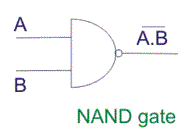During the operation of the NAND gate, the inputs are first going through AND gate and after that, the output gets reversed, and we get the final output. Now we will look at the truth table of NAND gate.

We will consider the truth table of the above NAND gate i.e. a two-input NAND gate. The two inputs are A and B.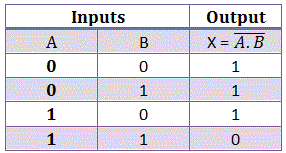Now we will see how this gate can be used to make other gates.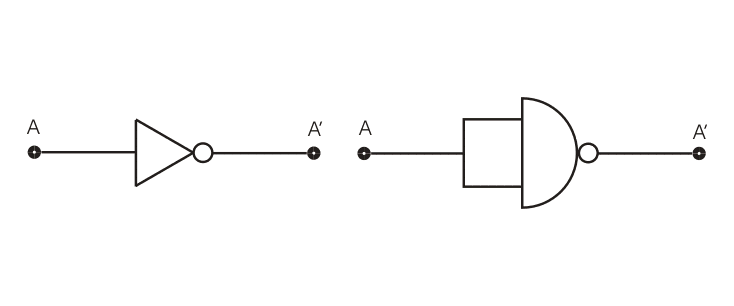This is the circuit diagram of a NAND gate used to make work like a NOT gate, the original logic gate diagram of NOT gate is given besides the circuit diagram below.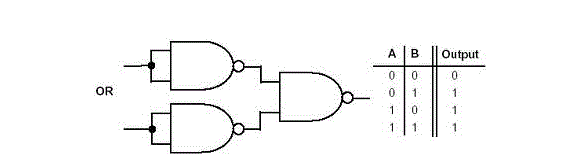The above diagram is of an OR gate made from combinations of NAND gates, arranged in a proper manner. The truth table of an OR gate is also given beside the diagram.

Now we will see the design of an AND gate from NAND gates.The above diagram is of an AND gate made from NAND gate. So we can see that all the three basic gates can be made by only using NAND gates, that’s why this gate is called Universal Gate, and it is appropriate.

## NOR Gate As A Universal Gate

We have seen how the NAND gate can be used to make all the three basic gates by using that alone. Now we will discuss the same in case of NOR gate.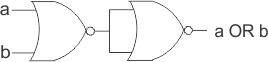The above diagram is of an OR gate made by only using NOR gates. The output of this gate is exactly similar to that of a single OR gate. We can see the circuit arrangement of OR gate using, NOR gate is similar to that of AND gate using NAND gates.The above diagram as the name suggests is of AND gate using only NOR gate, again we can see that the circuit diagram of AND gate using only NOR gate is exactly similar to that of OR gate using only NAND gates. Now we will finally see how we can make a NOT gate by using only NOR gates.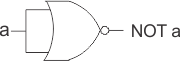The above diagram is of a NOT gate made by using a NOR gate. The circuit diagram is similar to that of NOT gate made by using only NAND gate.

So, from the above discussion, it is clear that all the three basic gates (AND, OR, NOT) can be made by only using NOR gate. And thus, it can be aptly termed as Universal Gate.

Want To Learn Faster? 🎓
Get electrical articles delivered to your inbox every week.
No credit card required—it’s 100% free.